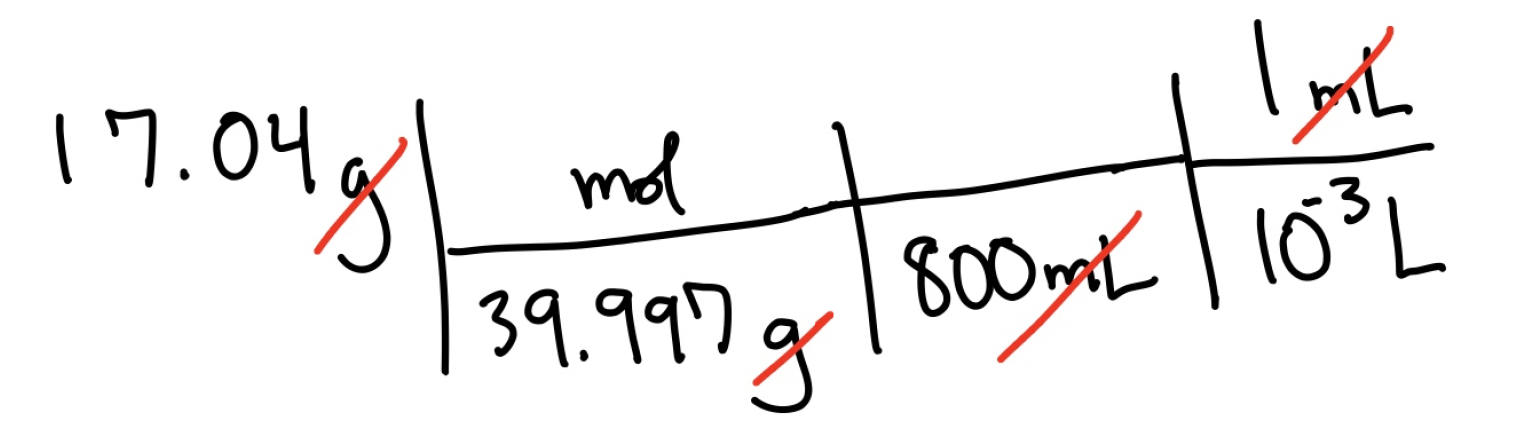Having trouble writing your personal statement?

MCAT Content / Stoichiometry / Metric Units Commonly Used In The Context Of Chemistry

### Metric Units Commonly Used in the Context of Chemistry

Topic: Stoichiometry

Important units used in the context of chemistry, especially stoichiometry, are grams, moles, liters, centimeters, and meters.

These metric units, also known as SI Units (International System of Units, recognized and used globally in science), are fundamental in calculating conversions and mathematical equations in chemistry. In chemistry, the use of these units is categorized under stoichiometry.

Stoichiometry is the study of the relationships between quantities of substances and, in the context of chemistry, helps us to utilize values for calculations that otherwise would be impossible without the conversion of units.

Grams measure the mass of a substance per one mole of the substance. On the periodic table, we can obtain the mass or molecular weight of an element. From the periodic table, we could define the mass of hydrogen is 1.008 grams per mole; moles are further defined in Avogadro’s Number.

Liters are units used to describe the volume of a gas or liquid.

Centimeters and meters can be used to describe the length and volume of an object.

To understand how all of these units can be used with one another, let us observe the conversion of NaOH from a volume in cm3 to its concentration (molarity) in an 800 mL solution.As covered in the Density topic, we understand that 1 mL is equal to one centimeter cubed, or cm3. We can convert the given volume of NaOH to grams by multiplying the volume by the density. Notice how units that are both on the top and bottom of the line effectively “cancel” out.From grams, the moles of NaOH can be obtained by dividing the mass by the molar mass. Because we want to know the concentration of NaOH in 800 mL, we can simply divide the moles by a volume (the final value will be in liters, as seen above). The final value will be in mol/L, or M.Hopefully this example displays how we can use standardized units to solve problems!

Practice Questions

Blood flow in the arteries

MCAT Official Prep (AAMC)

Chemistry Question Pack Passage 3 Question 10

Chemistry Question Pack Passage 3 Question 12

Chemistry Question Pack Passage 16 Question 87

Chemistry Question Pack Passage 18 Question 102

Key Points

• Units commonly used in chemistry include grams, moles, liters, centimeters, and meters. These units are also known as SI Units.

• When converting units, it is helpful to set up conversions so that units in the numerator (top) and denominator (bottom) of equations that are similar can cancel out.

Key Terms

stoichiometry: the study of the relationships between quantities of substances

SI units: International System of Units is the modern form of the metric system

molecular weight: the total mass of atoms in a molecule, represented by g/mol

mole: use to represent the amount of substance in a chemical reaction

avogadros number: the number of atoms in one mole of substance (6.02×1023)

Billing Information